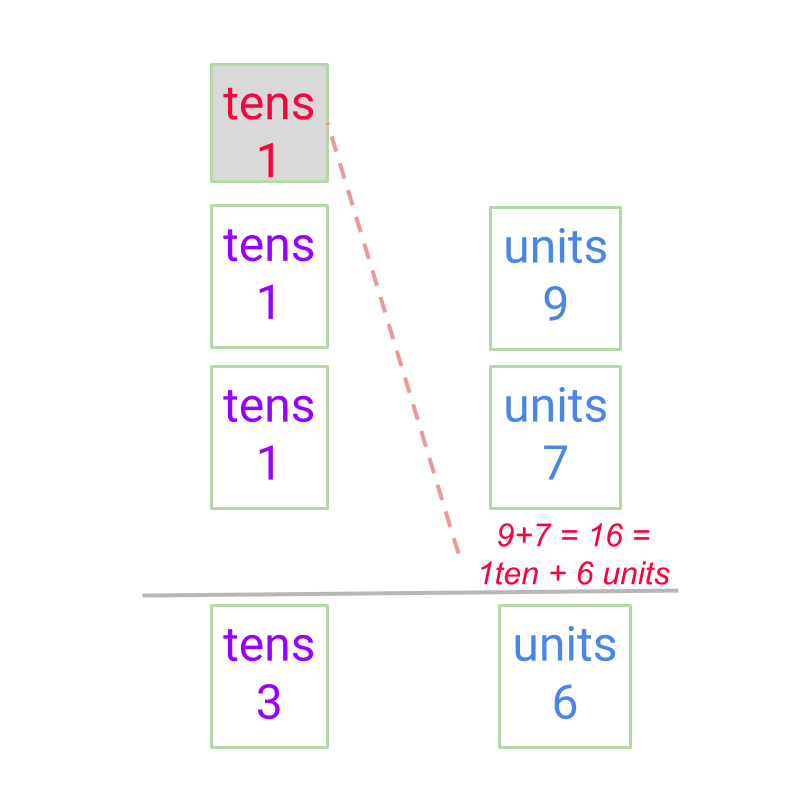maths > integers

what you'll learn...

overview

The addition in first principles was explained as combining two quantities and counting or measuring the combined quantity.

For integers, the positive and negative numbers are to be handled appropriately. By considering all the combination of signs in addends, a simplified procedure is derived.

regrouping

In whole numbers, we had studied the following.

Addition by Place-value with regrouping -- simplified procedure : Two numbers are added as per the following procedure:

•  the place-value positions are arranged units under units, $10$$10$s under $10$$10$s, etc.

•  the units are added and if the result has $10$$10$ numbers, then it is carried to the $10$$10$s place.

•  The addition is continued to the higher place-value position.The carry over is the simplification of combining $10$$10$ of a place value to a higher place value.

positive and negative

The terminology "received" and "given" symbolically refer to the positive and negative numbers of all applications.

For example, temperature ${3}^{\circ }$${3}^{\circ}$ above $0$$0$ and ${2}^{\circ }$${2}^{\circ}$ below $0$$0$ are referred as $\text{received:}3$$\textrm{\left(r e c e i v e d\right\rangle} 3$ and $\text{given:}2$$\textrm{\left(g i v e n\right\rangle} 2$ respectively. The same in integers is $3$$3$ and $-2$$- 2$.

Integers are directed whole numbers.

$\text{received:}3$$\textrm{\left(r e c e i v e d\right\rangle} 3$ is also referred as \text{aligned:}3$\textrm{\left(a l i g \ne d\right\rangle} 3$, meaning the magnitude given by the number is aligned to the chosen direction.

$\text{given:}2$$\textrm{\left(g i v e n\right\rangle} 2$ is also referred as $\text{opposed:}2$$\textrm{\left(o p p o s e d\right\rangle} 2$, meaning the magnitude given by the number is opposed to the chosen direction.

For example, temperature ${3}^{\circ }$${3}^{\circ}$ above $0$$0$ and ${2}^{\circ }$${2}^{\circ}$ below $0$$0$ are referred as \text{aligned:}3$\textrm{\left(a l i g \ne d\right\rangle} 3$ and $\text{opposed:}2$$\textrm{\left(o p p o s e d\right\rangle} 2$ respectively. The same in integers is ${3}^{\circ }C$${3}^{\circ} C$ and $-{2}^{\circ }C$$- {2}^{\circ} C$.

simplify

$4+3=7$$4 + 3 = 7$

By first principles, $\text{received:}4$$\textrm{\left(r e c e i v e d\right\rangle} 4$ and $\text{received:}3$$\textrm{\left(r e c e i v e d\right\rangle} 3$ together is $\text{received:}7$$\textrm{\left(r e c e i v e d\right\rangle} 7$.

$4+\left(-3\right)=1$$4 + \left(- 3\right) = 1$

By first principles, $\text{received:}4$$\textrm{\left(r e c e i v e d\right\rangle} 4$ and $\text{given:}3$$\textrm{\left(g i v e n\right\rangle} 3$ together is $\text{received:}1$$\textrm{\left(r e c e i v e d\right\rangle} 1$.

This addition is equivalent to whole number subtraction $4-3=1$$4 - 3 = 1$.

$4+\left(-7\right)=\left(-3\right)$$4 + \left(- 7\right) = \left(- 3\right)$

By first principles, $\text{received:}4$$\textrm{\left(r e c e i v e d\right\rangle} 4$ and $\text{given:}7$$\textrm{\left(g i v e n\right\rangle} 7$ together is $\text{given:}3$$\textrm{\left(g i v e n\right\rangle} 3$.

This addition is equivalent to whole number subtraction $7-4=3$$7 - 4 = 3$, with the result modified to the sign of larger absolute value.

$\left(-4\right)+\left(-3\right)=-7$$\left(- 4\right) + \left(- 3\right) = - 7$

By first principles, $\text{given:}4$$\textrm{\left(g i v e n\right\rangle} 4$ and $\text{given:}3$$\textrm{\left(g i v e n\right\rangle} 3$ together is $\text{given:}7$$\textrm{\left(g i v e n\right\rangle} 7$.

This addition is equivalent to whole number addition $4+3=7$$4 + 3 = 7$, with result modified to the sign of the two addends.

Summary of integer addition illustrative examples:

•  $4+3=7$$4 + 3 = 7$ : To $\text{received:}4$$\textrm{\left(r e c e i v e d\right\rangle} 4$ another $\text{received:}3$$\textrm{\left(r e c e i v e d\right\rangle} 3$ is put-in

•  $4+\left(-3\right)=1$$4 + \left(- 3\right) = 1$ : To $\text{received:}4$$\textrm{\left(r e c e i v e d\right\rangle} 4$ another $\text{given:}3$$\textrm{\left(g i v e n\right\rangle} 3$ is put-in

•  $-4+3=-1$$- 4 + 3 = - 1$ : To $\text{given:}4$$\textrm{\left(g i v e n\right\rangle} 4$ another $\text{received:}3$$\textrm{\left(r e c e i v e d\right\rangle} 3$ is put-in

•  $4+\left(-7\right)=-3$$4 + \left(- 7\right) = - 3$ : To $\text{received:}4$$\textrm{\left(r e c e i v e d\right\rangle} 4$ another $\text{given:}7$$\textrm{\left(g i v e n\right\rangle} 7$ is put-in

•  $-4+\left(-3\right)=-7$$- 4 + \left(- 3\right) = - 7$ : To $\text{given:}4$$\textrm{\left(g i v e n\right\rangle} 4$ another $\text{given:}3$$\textrm{\left(g i v e n\right\rangle} 3$ is put-in

Based on this, the addition is simplified as

•  +ve + +ve is whole number addition of absolute values

•  +ve + -ve is subtraction as given below
absolute values of the two numbers are compared
subtract the number of smaller absolute value from the number of larger absolute value. The difference is the absolute value of the result.
the sign of result is the sign of the number with larger absolute value

•  -ve + -ve The result is sum of absolute values with negative sign.

The addition or subtraction of absolute values in the procedure are detailed in addition by place-value and subtraction by place-value.

examples

Find the sum of $-6$$- 6$ and $0$$0$.
The answer is "$-6$$- 6$"

Find the sum of $11$$11$ and $-140$$- 140$
The answer is "$-129$$- 129$"

Find the sum of $2$$2$ and $-2$$- 2$
The answer is "$0$$0$"

Find the sum of $-2345$$- 2345$ and $-889$$- 889$
The answer is "$-3234$$- 3234$"

summary

Addition of Integers (Simplified Procedure) : Two numbers with signs positive or negative are added as follows.

•  +ve $+$$+$ +ve is whole number addition
•  +ve $+$$+$ -ve is subtraction as given below
•  -ve $+$$+$ -ve is addition of absolute values with negative sign.